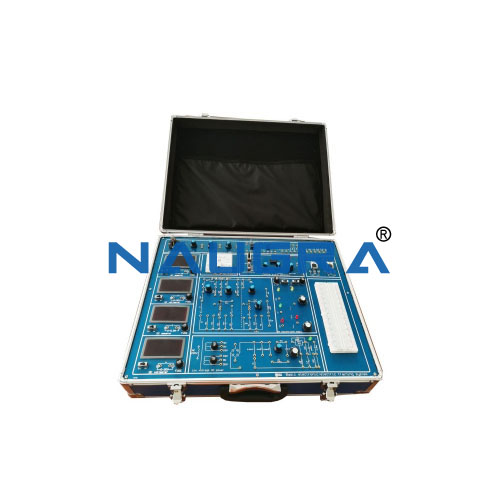# Basic Electricity Plug-in Training Kit## Basic Electricity Plug-in Training Kit

Lab Catalog Supply Code: NED-18-06-18-0023

Basic Electricity Plug-in Training Kit
The Kit Should Cover the Following Training Contents  (like or better than):
• Assembly of simple circuits
Use of voltmeters and ammeters.
Ohm’s law
Series and parallel connection of resistors
Voltage dividers with and without loads
Light bulb characteristics
Voltage dependent resistors (VDRs)
Negative temperature coefficient thermistors (NTCs)
Positive temperature coefficient thermistors (PTCs
Light-dependent resistors (LDRs)
Capacitors in DC circuits
Relay circuits
Generating AC
Characteristics of AC
Resistors in AC circuits
Capacitors in AC circuits
Series connections of resistors and capacitors (RC)
parallel connections of resistors and capacitors (RC)
Coils (inductors) in AC circuits
Series connections of resistors and inductors (RL)
Parallel connections of resistors and inductors (RL)
Series connections of resistors, inductors and capacitors (RLC)
Parallel connections of resistors, inductors and capacitors (RLC)
Series compensation
Parallel compensation